# A Tweakable Image Encryption Algorithm Using an Improved Logistic Chaotic Map

A Tweakable Image Encryption Algorithm Using an Improved Logistic Chaotic Map

Electronics Research Laboratory, University of 20 August 1955, Skikda 21000, Algeria

Laboratory of Systems Analysis and Signals (LASS), University of Mohamed Boudiaf, M’Sila 28000, Algeria

Conservatoire National des Arts et Métiers, 292 Rue Saint-Martin, Paris Cédex 03 F-75141, France

Corresponding Author Email:
Page:
407-417
|
DOI:
https://doi.org/10.18280/ts.360505
19 May 2019
|
Accepted:
20 August 2019
|
Published:
25 November 2019
| Citation

OPEN ACCESS

Abstract:

This paper aims to improves the chaotic behavior of classical logistic chaotic map for image encryption. First, this classical technique was enhanced, and the effectiveness of the improved technique was verified by the bifurcation diagram and Lyapunov exponent, in comparison to the classical technique. On this basis, an efficient tweakable image encryption algorithm was proposed to protect the security of digital image transmission. The proposed algorithm adopts a confusion-diffusion architecture. With the aid of the tweak, each original image is encrypted as multiple different images using the same secret key-stream. The experimental results prove that the proposed algorithm, despite its simplicity, can withstand several types of attacks through image encryption. The research results shed new light on the data security in the transmission of digital images.

Keywords:

image encryption, chaos, logistic map, tweakable

1. Introduction

In today’s information technology systems, it is very important to protect exchanged data of which image content is an overwhelming part. For this reason, image encryption has become an urgent challenge and high concern which has attracted many researchers in recent years. Several image encryption schemes have been developed using diverse techniques such as quantum theory [1, 2], DNA coding [3, 4], chaos theory [5-12], and very recently Hua et al.  have developed an image encryption algorithm with Josephus shuffling and filtering diffusion, to get better diffusion effectiveness. The introduction of chaos in image encryption techniques is mainly due to the good features it offers for image data protection, such as its extremely high sensitivity dependence on initial conditions and control parameters, nonlinearity, ergodicity and random-like behaviors . In addition, the security of an image encryption scheme that uses a chaotic map output sequences usually depends on two parts, namely, the permutation and diffusion processes [1-5]. In the permutation process, the pixel locations of the images are altered to remove the redundancies and break the high correlation among adjacent pixels, while in the diffusion process, the image pixel values are changed. Some encryption schemes iterate these processes to increase the effectiveness of encryption, many chaos-based image encryption algorithms have been recently proposed to prevent unauthorized access, such as the work of Zhang and Wang , in which they proposed a multiple-image encryption algorithm based on mixed image element and permutation. Zahmoul et al.  proposed an image encryption based on new Beta chaotic maps, where these maps have been used to generate chaotic sequences which are used in the encryption scheme. Herbadji et al.  suggested a color image encryption scheme based on enhanced logistic chaotic map. Abanda and Tiedeu  introduced an image encryption by chaos mixing. Hua et al.  have proposed a cosine-transform-based chaotic system (CBCS), where they used two classical chaotic maps, this system can generate pseudo-random streams with complex dynamical conducts, and furthermore they proposed an image encryption scheme using CBCS. Liu et al.  suggested an image encryption system that is adopted on strong chaotic maps and one-time keys. Liu and Wang  proposed a color image encryption scheme employing spatial bit-permutation and high-dimensional chaotic maps. Liu et al.  developed an image encryption algorithm using DNA encoding and chaos maps, the secret key is produced using the image, the one to be encrypted and a common key. Wang et al. have designed an image block encryption algorithm based on perceptron model with a neural network and the high dimension of Lorenz system .

In practical communication systems, fast encryption in the real-time plays an important role, Wang et al.  suggested a fast image encryption scheme by using parallel diffusion technique, the resulted  system greatly enhances the encryption efficiency, where the data volume to be protected is large, in this technique it is  needed to use the parallel computation techniques to enhance the efficiency

In the image encryption based on chaotic maps, the encryption algorithm security depends on the characteristic of the chaotic maps and the structure of the algorithm [16, 17], thus a better chaotic map distribution is required. However, classical chaotic systems such as logistic and quadratic maps suffer many weaknesses, not the least is the limited range of their chaotic conducts, in addition to non-uniform data distribution of the generated chaotic sequences . Chaotic maps with weak chaotic behaviors can make the cryptosystems vulnerable to attacks and can be easily broken. In recent years several works available in the literature have suggested to overcome the disadvantages of these chaotic maps by improving the properties of chaotic distribution for better performance and effectiveness of image encryption algorithms [19, 20]. Hua et al.  suggested a sine chaotic model (SCM) to improve the chaos complexity of existing chaotic range of (1-D) chaotic maps. Hua et al.  have proposed a sine-transform-based chaotic system (STBCS) to produce an effective one-dimensional chaotic map, where they performed a sine transform to the combination of the outputs of two existing chaotic maps.

In order to disband the aforementioned drawbacks, this work suggests an enhanced logistic map and evaluates its performance. The analysis results of the bifurcation diagram of this enhanced chaotic system and Lyapunov exponent show that it has good chaotic performance. Additionally, the chaotic performance is analyzed by a new proposed chaotic image encryption algorithm. To test the applications of the enhanced logistic map in image encryption, a novel tweakable image encryption algorithm consisting of a confusion-diffusion architecture has been proposed. The scheme includes two runs of the diffusion and confusion process. The concept of tweak encryption is used to guarantee the variability of the suggested technique. Thus, with the use of the tweak, an original image will be encrypted to different encrypted-images by using the same secret key-stream, because by changing the tweak it is less costly and faster than changing the key of the suggested scheme .Therefore, the suggested method can successfully withstand the chosen plaintext attack. Thus, the goal of the tweak is to guarantee the variability. Furthermore, the tweaks take additional parameters as input in addition to the plain image and the secret key, where these parameters permit to control the value of the outputted encrypted image without affecting the secret keys . Thus, the suggested algorithm breaks the limitation of the algorithm based on one-time keys. Moreover, the proposed scheme can encrypt many images securely and speedily using the same key. The experimental results demonstrate that the algorithm is simple, efficient and has good execution in image encryption and has also the ability to withstand several attacks.

The motivation of this work is to analyze the shortcomings of the Logistic chaotic map in term of pseudo-random sequences and also to improve their chaotic behavior in order to be suitable for image encryption. Based on this improved chaotic map, an efficient images encryption algorithm is proposed in this paper, in order to ensure the security requirements of digital image transmission.

The paper is organized as follows. The second section briefly displays the effectiveness of existing Logistic map. The third section reviews an improved logistic chaotic system by utilizing the aforementioned existing classical logistic chaotic map and explain its accuracy. The fourth section suggests a new tweakable image encryption scheme. The fifth Section displays the simulation results and analysis. Finally, section 6 presents the conclusion.

2. Analysis of Logistic Map

Logistic map is one of the most famous 1D chaotic maps, which has simple and classic dynamical nonlinear equation with complex chaotic behaviors, it can be described by the following equation .

$X _ { n + 1 } = r X _ { n } \left( 1 - X _ { n } \right)$    (1)

where, r is the control parameter with range of $\mathrm { r } \in [ 0,4 ]$ and Xn is the output chaotic sequence.

2.1 Bifurcation diagram

The diagram of bifurcation is the study of the chaotic system as a mathematical function of the values of the parameters of control . From its bifurcation diagram presented in Figure 1(a), two defects can be noticed in this chaotic map: 1) It has a limited ambit of chaos and, 2) as demonstrated in Figure 1(a), Figure 2(a), the chaotic ambit exists only within [3.57, 4]. When the parameter r do not belong to this range, it cannot be considered to have a chaotic behavior and non-uniform distribution of the output chaotic sequences that affected the distributions of encrypted image data and the performance of encryption system.

## a1.png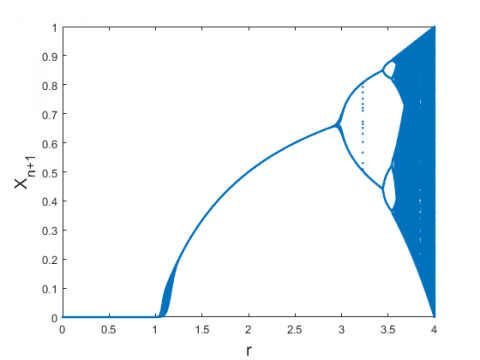(a)

## 1b.png(b)

Figure 1. The bifurcation diagrams of the (a) Classical logistic map; (b) The improved logistic map

## 2a.png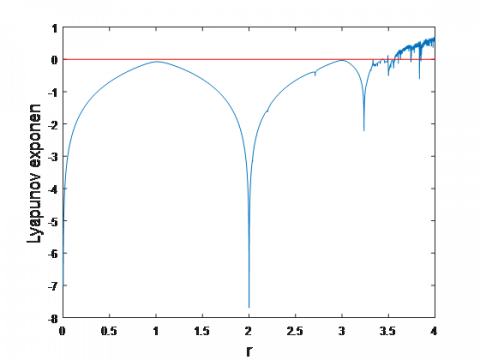(a)

## 2b.png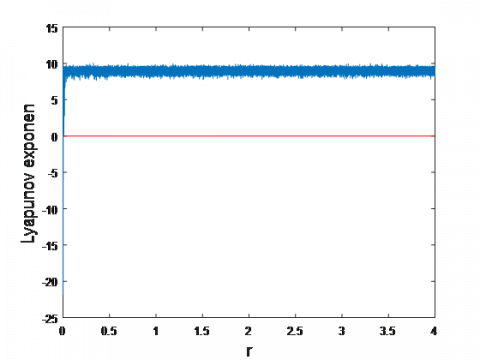(b)

Figure 2. The Lyapunov exponent of the (a) Classical logistic map; (b) The improved logistic map

2.2 Lyapunov exponent

The Lyapunov exponent (LE) represents a quantitative measure of the sensitivity of the control parameters of chaotic maps . Mathematically it can be represented as:

$\lambda = \lim _ { n \rightarrow \infty } \frac { 1 } { n } \sum _ { i = 0 } ^ { n - 1 } \ln \left| f ^ { \prime } x _ { i } \right|$       (2)

If λ is positive, this means that the system has good chaotic behaviors and, the larger the value of the quantity λ, the better is the LE. From Figure 2(a), it can be seen that the logistic map is chaotic only when $\mathrm { r } \in [ 3.57, 4 ]$ and the maximum Lyapunov exponent of the logistic map is 0.6720.

3. The Proposed Improved Logistic Map

In this section, logistic map is enhanced to solve the aforementioned defects. The formula of the improved logistic map is described as follows.

$X _ { n + 1 } = r \left( 2 ^ { k } \times X _ { n } \right) \left( 1 - \left( 2 ^ { k } \times X _ { n } \right) \right) \bmod 1$    (3)

where, Xn in Eq. (1) is replaced with the term $\left( 2 ^ { \mathrm { k } } \times \mathrm { X } _ { \mathrm { n } } \right)$, also the 'mod' operation is applied to assure that generated chaotic streams are within range of [0, 1] and the map has good chaotic performance, when kis within the range [2, 10], this range has been proved in the experiment. The improved chaotic map is examined by the bifurcation diagram and by Lyapunov exponent at the value of k= 8.

3.1 Analysis of the improved logistic map

Figures 1(b) and 2(b) show the bifurcation diagram and the Lyapunov Exponent of the improved chaotic map. The chaotic behaviors of this improved system exist in the whole ambit of the control parameters and it is quite bigger than its seed map. Thus, the improved map has good chaotic performance and is very proper in ciphering schemes.

3.2 Randomness

In the chaos-based image encryption schemes, it is significant to evaluate the goodness of randomness of the sequences generated by the improved chaotic map to ensure that they are proper for encryptions, for this reason we have performed NIST test.

The NIST statistical set of tests contain 15 statistical runs to test randomness property of a binary sequence . For each test, If the P value is bigger than 0.01, this shows that the binary sequence has successfully passed the tests, while the opposite shows non randomness . The results in Table 1 show that the improved logistic map has passed all the NIST tests successfully. Thus, the random numbers generated by this improved chaotic map are ready for encryptions.

Table 1. Nist SP800-22 randomness results for the improved logistic map

 Test P_values FREQUENCY 0.0367978346837154 Block frequency 0.379761079097109 RUNS 0.25610508084267 LONGEST RUNS 0.892791595823537 RANK TEST 0.382355232911561 FFT 0.0128873729555428 Non-overlapping-templates 0.451951905074747 Overlapping-templates 0.854994085337196 Universal 0.0598171684079449 Linear-complexity 0.3071407630330160 Approximate entropy 0.501974855003042 CUMULATIVE SUMS 0.061082359059655 Random-excursions variant (x=2) 0,887616419296518 Serial 1 0.433025725806859 Serial 2 0.370075060883015 RANDOM EXCURSIONS(x=-7) 0.987809436580346
4. Suggested Image Encryption Algorithm

In this section, we suggest a new tweakable image encryption algorithm, the proposed encryption algorithm uses 16 parameters, given as: (x0,1, x0,2, x0,3, x0,4, x0,5, x0,6, r1, r2, r3, r4, r5, r6, T1, T2, T3, T4) considered as the security key. The schema of the proposed encryption algorithm is illustrated in Figure 3. It has a two tours of encryption operation, in this algorithm every encrypted-pixel is not only related to the original pixel that generates it, but to all the other pixels. Therefore, a small change in any original image pixel leads to a totally different encrypted image. Furthermore, the concept of tweakable block cipher has been used to ensure the characteristic that will change the tweak to be less costly and faster than changing the key of the suggested scheme . In our proposed scheme, the tweaks (T1, T2) is of the first round and (T3, T4) is of the second round they are related to the plaintext image to be ciphered. Different images have different tweaks, (T1, T2) of first round and (T3, T4) of second round, therefore even if the attacker get the tweak (T1, T2, T3 and T4) and the key of encryption of some special chosen plaintext images, these tweaks cannot be applied to decrypt the ciphertext image which the attacker want to obtain. Thus, the goal of the tweak is grant changing without changing the key of encryption.

## 3.png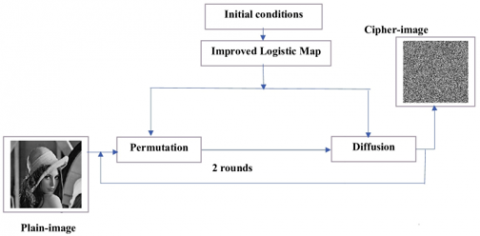Figure 3. Block diagram of proposed scheme

4.1 Encryption algorithm

Input: Plain image I with a size of W × H.

Output: The ciphered image C.

Step 1:Two different chaotic sequences are generated

S = {S1, S2… SSW}, Z = {Z1, Z2,..... ZZH} of size W×H, by using the Eq. 3 with the initial values $\left( x _ { 0,5 } , r _ { 5 } \right) , \left( x _ { 0,6 } , r _ { 6 } \right)$, respectively.

Step 2: In order to break the intense correlation among neighboring pixels, we propose a new permutation algorithm with the ability to simultaneously change the row and column of an image in the same process. Two tours of a permutation can obtain an excellent confusion result in theory. Hence, our proposed approach uses two encryption rounds to obtain high-security. The procedure of proposed permutation algorithm can be described as follows:

1. Two random sequences,X=(X1, X2...... XH) of length H and Y = (Y1, Y2...... YW) of length W are generated by using equations 3, with the initial values $\left( x _ { 0,1 } , r _ { 1 } \right) , \left( x _ { 0,2 } , r _ { 2 } \right)$ respectively.
2. X and Y are sorted respectively to obtain two index sequences, I and J.
3. Two random matrix, A of length H × 2 and B of length W × 2 are generated by using Eq.3, with the initial values $\left( x _ { 0,3 } , r _ { 3 } \right) , \left( x _ { 0,4 } , r _ { 4 } \right)$, respectively. These will be used to control the scan and permutation direction. Algorithm 3 displays the code of the proposed permutation process, and to better explain the process of the proposed permutation, a numerical example with an image of size 8× 8 is given in Figure 5 and Figure 6. The obtained image is reshaped into one vector, P”’=(p1, p1 ......pW×H×1).

Step 3: The diffusion process of the proposed scheme is detailed in Algorithm 1 and Figure 4, which takes three inputs: the secret keys, in the output a cipher-image C = {C1, C2, ...C1×W×H} is produced and two tweak T1 and T2.

Algorithm 1. Diffusion

 1 input: Permuted_image: P”’; Secret_keys: x0,2,u0,2, k2, x0,3,u0,3, k3 2 output: Encrypted_image: C, Tweak T1,T2,T3 and T4 3 Secret_keysare used to obtain a chaotic sequence X and Z of size H × W, Z and X are converted to a sequence of integers values, by following equation 4 Z(i)=floor (Z(i) × 1015), X(i)=floor (X(i) × 1015) 5 $\mathrm { n } \leftarrow \mathrm { H } \times \mathrm { W } , \mathrm { t } 1 \leftarrow$ rand $(),$ where $\mathrm { t } 1 \in [ 0,255 ]$ 6 $\mathrm { T } 1 \leftarrow P ^ { \prime \prime \prime } \mathrm { n } ^ { \prime \prime } \oplus \mathrm { t } 1$ 7 $G _ { n + 1 } \leftarrow \mathrm { T } 1$ 8 $\mathrm { for } \mathrm { i } \leftarrow \mathrm { n }$ to 1 9 $G _ { i } \leftarrow P ^ { \prime \prime \prime } _ { i } \oplus Z _ { i } \oplus G _ { i + 1 }$ 10 $\quad$ end 11 $\mathrm { t } 2 \leftarrow$ rand $() ,$ where $\mathrm { t } 2 \in [ 0,255 ]$ 12 $\mathrm { T } 2 \leftarrow P ^ { \prime \prime \prime } , \oplus \mathrm { t } 2$ 13 $\mathrm { c } _ { 1 } \leftarrow \mathrm { T } 2 \oplus G _ { 1 }$ 14 $C _ { 1 } \leftarrow \left( c _ { 1 } + X _ { 1 } \right) \mathrm { mod } 256$ 15 for $\mathrm { i } \leftarrow \left( c _ { 1 } + X _ { 1 } \right) \mathrm { mod } 256$ 16 $c _ { i } \leftarrow G _ { i } \oplus C _ { i - 1 }$ 17 $\quad C _ { i } \leftarrow \left( c _ { i } + X _ { i } \right) \bmod 256$ 18 end 19 The encrypted $\mathrm { C }$ is Reshaped into $2 \mathrm { D }$ matrin $\mathrm { W } \times \mathrm { H }$

## 4.pngFigure 4. The proposed method of tweak-based diffusion scheme using 4 pixels

4.2 Decryption algorithm

The decryption process is the reverse process of encryption. The reverse process of diffusion has been explained in Algorithm 2.

Algorithm 2. Inverse_diffusion

 1 input: Encrypted_image C; Secret_keys: x0,2,u0,2, k2, x0,3,u0,3, k3,Two tweak T1,T2 2 output: Permuted_image: P Secret_keysare used to obtain a chaotic sequence X and Z of size H × W, Z and X are converted to a sequence of integers values, by following equation: 3 Z(i)=floor(Z(i) ×1015)), X(i)=floor(X(i) ×1015)) 4 n←H×W 5 $G _ { 1 } \leftarrow c _ { 1 } \oplus \mathrm { T } 2$ 6 $c _ { 1 } \leftarrow \left( C _ { 1 } - X _ { 1 } \right) \mathrm { mod } 256$ 7 for $\mathrm { i } \leftarrow 2$ to $\mathrm { n }$ 8 $\mathrm { c } _ { i } \leftarrow \left( C _ { i } - X _ { i } \right) \mathrm { mod } 256$ 8 $G _ { i } \leftarrow c _ { i } \oplus C _ { i - 1 }$ 10 end 11 $P _ { n } \leftarrow G _ { n } \oplus Z _ { n } \oplus \mathrm { T } 1$ 12 for $\mathrm { i } \leftarrow \mathrm { n } - 1$ to 1 13 $P _ { i } \leftarrow G _ { i } \oplus Z _ { i } \oplus G _ { i + 1 }$ 14 end

Algorithm 3. Permutation

 1 Input: A, B, I, J, P. 2   Output: Permuted greyscale image P”’ 3    k←H 4       for i← 1 to W do 5          if A(I(i), 1) ≥ A(I(i), 2) then // 6           for j ← 1 to H do 7                       The row I(i) is permuted from the left to right by using the following: 8                        P’(I(i), j) ← P (i, j) 9            End 10        Else 11           for j ← 1 to H do 12                   The row I(i) is permuted from the right to left by using the following: 13                      P’(I(i), k) ← P (i, k) 14                     K ← k − 1; 15               End 16 End 17 K ← H 18         End 19    The second round of permutation 20     P”← rot90(P’) 21    k ← W 22   for i← 1 to H do 23        if B(J(i), 1) ≥ B(J(i), 2) then 24            for j ← 1 to W do 25                  The row J(i) is permuted from the left to right by using the following: 26                  P”’(J(i), j) ← P”(i, j) 27            End 28          Else 29             for j ← 1 to W do 30              The row J(i) is permuted from the right to left by using the following: 31                P”’(J(i), k) ← P”(i, k) 32                K ← k − 1; 33             End 34          End 35    K ← W 36   End 37  P← P”’

## 5.png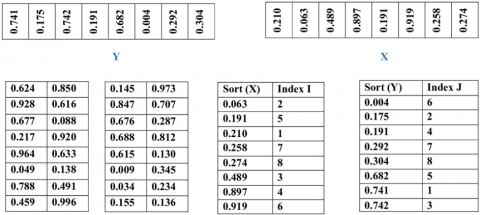Figure 5. An example of generating chaotic sequences: (a) generating two 1d index matrices I and J; (b) generating two random matrices A and B

## 6.png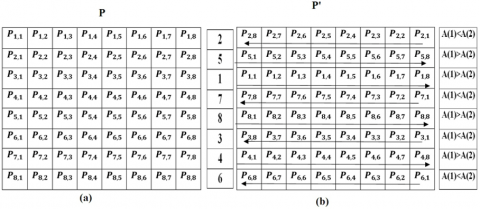## 6b.png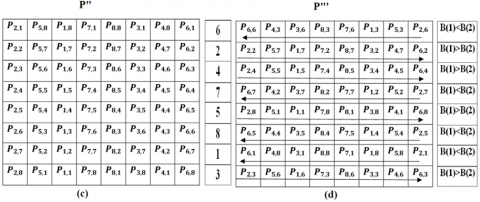Figure 6. An example of proposed permutation: (a) pixels in original image P; (b) permutation to P’ using I (c) image rotated 90 degrees counter clockwise in the second round of encryption;(d) permutation to P” using j

5. Experimental Results

In this section, the performance of the suggested algorithm will be discussed through the obtained results. In addition, some types of test will be used to show the superiority of the proposed encryption method. The quantities to be measured are: NPCR, UACI, the correlation analysis, key space, key sensitivity and information entropy evaluation, randomness of encrypted-images. To further explain the effectiveness of the suggested method, it has been compared with the following advanced image encryption algorithms: [3, 27-30].

5.1 Key space analysis

In order to ensure that brute force attack is infeasible, key space should be greater than 2100 . The secret keys that is used in our proposed scheme are summarized as: 1- The control parameters r1, r2, r3, r4, r5, r6. 2- The initial values x0,1, x0,2, x0,3, x0,4, x0,5, x0,6 and four tweakT1, T2, T3 and $\mathrm{T} 4 \in[0,255]$, the space of every initial value is 1015, so the key space of our suggested scheme is 4×256×1012×15. Table 2 lists the key space comparison of our proposed algorithm with some advanced image encryption algorithms [3, 25, 26, 27, 28]. Therefore, it is large enough to resist to the brute force attack and the suggested encryption algorithm is better to withstand brute-force attack.

Table 2. Comparison with some algorithms in key space

$\begin{array} { c c } \hline \text { Algorithm } & { \text { Key space } } \\ \hline \text { Our } & { 4 \times 256 \times 10 ^ { 12 \times 15 } \approx 2 ^ { 600 } } \\ { \text { Ref.  } } & { \approx 2 ^ { 256 } } \\ { \text { Ref. } } & { \approx 2 ^ { 256 } } \\ { \text { Ref. } [ 3 ] } & { \approx 2 ^ { 400 } } \\ { \operatorname { Ref } . [ 30 ] } & { \approx 2 ^ { 320 } } \\ { \operatorname { Ref } . [ 30 ] } & { \approx 2 ^ { 320 } } \\ \hline \end{array}$

5.2 The histogram analysis

The image histogram illustrates the number of pixels of every gray level . To withstand statistical attacks, the histogram should be fairly uniform. Figure 7 and 8 display the histograms of the plain-images and the histograms of their cipher-images. From Figure 7 and 8, the histogram of the cipher-images is fairly uniformed and ﬂat distribution, so that it is enough to makes statistic attacks infeasible.

## 7.png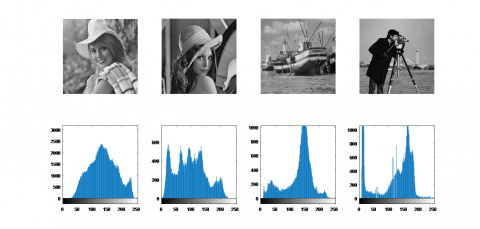Figure 7. Original images of ‘Elaine’, ‘Lena’, ‘Boat’, ‘Cameraman’ and their histograms

## 8.png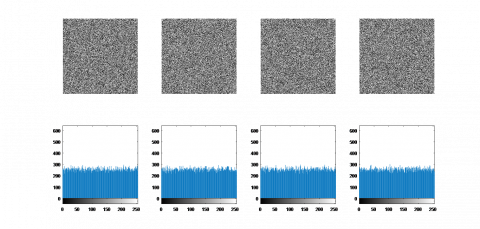Figure 8. Encrypted images of ‘Elaine’, ‘Lena’, ‘Boat’, ‘Cameraman’ and their histograms respectively (from left to right)

Table 3. Variances of histograms of plain images and their encrypted images

 Image Original Encrypted Ref.  Cameraman 11097.33 255.44 266.82 Baboon 65912.54 268.65 271.65 Boat 96083.68 245.90 256.03

The variance of a histogram can quantitatively evaluate the uniformity of pixels values of ciphered images, the lower the variance value is, the higher the uniformity of the encrypted image . The variance of histograms is formulated as follows:

$\operatorname { Var } ( Y ) = \frac { 1 } { n ^ { 2 } } \sum _ { i = 1 } ^ { n } \sum _ { j = 1 } ^ { n } \frac { 1 } { 2 } \left( y _ { i } - y _ { j } \right) ^ { 2 }$    (7)

where, Y represents the vector of the histogram values, and yi and yj considered as the numbers of pixels which gray values are equal to i and j, respectively. Table 3 lists the values of histogram variance of plain images and its corresponding encrypted images, from one can be seen that the variance values of the histograms of plain images are larger than the variance values of histograms of encrypted images that are small. These results prove that the proposed scheme has uniformly distributed the distributions of histograms of encrypted images, and it can be seen that the obtained variance values by the proposed algorithm are smaller than algorithm . Thus, the proposed scheme is strong enough to withstand statistical attacks.

5.3 Information entropy analysis

Entropy is the most signiﬁcant characteristic to measure the unpredictability and randomness measurements of information, the ideally entropy close to 8 for greyscale image . Entropy of image I is deﬁned as:

$E ( m ) = - \sum _ { i = 0 } ^ { 255 } \operatorname { Pr } ( m i ) \log _ { 2 } \operatorname { Pr } ( m i )$     (8)

where, Pr(mi) denotes the probability of symbol mi. The Information Entropy values of different encrypted image by using our scheme are listed in Table 4 and it refers that the entropy of the proposed encrypted images is all close to ideally entropy 8 and they are all greater than the Information Entropy values obtained by Wang et al. , Ramadan et al. , and Wang et al. , it is observed that our scheme is better than the schemes including the ones suggested by Wang et al. , Ramadan et al. , and Wang et al. , therefore the proposed encryption method is secure up on the entropy attack.

Table 4. Information entropy analysis of various images

 Image Original Proposed Ref.  Ref.  Ref.  Lena 7.5691 7.9976 7.9975 7.9975 7.9970 Boat 7.1913 7.9971 7.9969 7.9974 7.9971 Cameraman 7.6879 7.9972 7.9971 7.9970 7.9972 Baboon 7.3579 7.9994 7.9974 7.9973 7.9969
5.4 The correlation coefficients

In digital images, every pixel has a strong correlation with its close pixels either in, vertical, diagonal or horizontal direction. Therefore, an efﬁcient cryptosystem should encrypt the images with very weakly correlation in the neighboring pixels. The correlation values can be calculated by the following equation :

$\operatorname { corr } = \frac { \operatorname { cov } ( \mathrm { x } , \mathrm { y } ) } { \sqrt { \mathrm { D } ( \mathrm { x } ) \mathrm { D } ( \mathrm { Y } ) } }$      (9)

where, X and Yare the sets composed of N pixel gray values, $\mathrm { xi } \in \mathrm { X }$ and $\mathrm { yi } \in \mathrm { Y }$, are two adjacent pixels,

$\mathrm { E } ( \mathrm { X } ) = \frac { 1 } { \mathrm { N } } \sum _ { \mathrm { i } = 1 } ^ { \mathrm { N } } \mathrm { x } _ { \mathrm { i } }$       (10)

$\mathrm { D } ( \mathrm { X } ) = \frac { 1 } { \mathrm { N } } \sum _ { \mathrm { i } = 1 } ^ { \mathrm { N } } \left[ \mathrm { x } _ { \mathrm { i } } - \mathrm { E } ( \mathrm { X } ) \right]$      (11)

$\operatorname { cov } ( \mathrm { X } , \mathrm { Y } ) = \frac { 1 } { \mathrm { N } } \sum _ { \mathrm { i } = 1 } ^ { \mathrm { N } } \left[ \mathrm { x } _ { \mathrm { i } } - \mathrm { E } ( \mathrm { X } ) \right] \left[ \mathrm { y } _ { \mathrm { i } } - \mathrm { E } ( \mathrm { Y } ) \right]$     (12)

The correlations among neighboring pixels at vertical, diagonal or horizontal of the plain image and its corresponding encrypted image are shown in Figure 9. The correlation coefficients according to all directions of some images are listed in Table 5. It can be seen that the encrypted image is very close to 0. Furthermore, we have compared our proposed algorithm to the one used in Ref. [3, 5, 25, 26, 27, 28], ours has the smallest correlation values in all directions therefore, the proposed cryptosystem protects the images against statistical attacks.

Table 5. Coefficient correlation analysis

$\begin{array} { c c c c c c c } \hline \text { Direction } & { \text { Plain-lmage } } & { \text { Cipher-finage } } & { \text { Ref. } [ 28 ] } & { \text { Ref. } [ 30 ] } & { \text { Ref. } [ 3 ] } & { \text { Ref. } [ 27 ] } & { \text { Ref. } [ 29 ] } \\ \hline \text { Direction } & { 0.9346 } & { - 0.0009 } & { 0.0016 } &{-0.0019}& { - 0.0014 } & { - 0.0017 } & { 0.0012 } \\ { \text { Horizontal } } & { 0.9693 } & { 0.0003 } & { - 0.0022 } & { 0.0019 } & { 0.0020 } & {0.0038}& { 0.0024 } \\ { \text { Vertical } } & { 0.9179 } & { - 0.0022 } & {-0.0004}&{ - 0.0038 } & { - 0.0007 } & { - 0.0006 } & { - 0.0006 } \\ \hline \end{array}$

## 9.png(a)                                          (b)

Figure 9. Correlation of adjacent pixels at different directions of Lena (256x256 pixels) (a) original image, (b) encrypted image

5.5 Key sensitivity analysis

## wbtilsb9dpcp4alxg.pngFigure 10. Results of the key sensibility of the decrypted image: (a) with correct key; (b) with r1 + 10-15; (c) with wrong x1, (d with wrong x2+10-15

In addition to the fact that the cryptosystem owns a key space suitable to resist to the brute force attack, also, the cryptosystem must be very sensitive to their keys [34-35], where even there is only a tenuous variance of 10-15  between the encryption and decryption keys leads to failure of decryption. To evaluate the sensibility of key of our proposed algorithm, we decrypt Lena image with tenuous variance in any of the parameters of the secret key, the results are shown in Figure 10. We note that for only a slight change of $10^{-15}$ , the decrypted image is completely different from the plain image, which proves that suggested scheme is very sensitive to its keys.

5.6 Tweak sensitivity

To verify the variability of the suggested scheme, the tweaks sensitivity has been tested, where the last significant bit of the tweak is changed in the decryption process with the same secret key that is used in the encryption process. Figure 11 shows the tweak sensitivity results of the suggested scheme, where in our algorithm a tiny change in tweaks leads to decryption fail. Therefore, the suggested scheme has so sensitive to the tiny tweak alteration.

## 11.jpg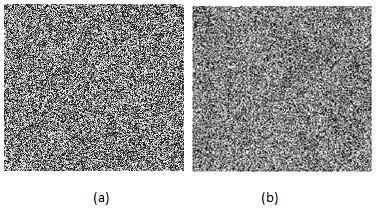Figure 11. Results of the Tweak sensitivity. (a) Decrypted image with wrong T1, (b) Decrypted image with wrong T2

5.7 Randomness tests

In order to confirm the randomness of encrypted images by the suggested scheme the NISTest of tests version is utilized. Table 6 shows that all ciphered images have passed all the NIST tests successfully. Therefore, the ciphered images that have been encrypted by the proposed scheme have good randomness features.

Table 6. NIST-2010 suite test results of sequence images

 Test Tested image Pepper Lena Boat Baboon FREQUENCY 0,424870 0,883921 0,939419 0,987234 Block frequency 0,228081 0,998861 0,419614 0,704799 RUNS 0,423341 0,505397 0,690625 0,866583 LONGEST RUNS 0,844824 0,844217 0,887254 0,048750 RANK TEST 0,784130 0,944003 0,212933 0,192916 FFT 0,287107 0,748072 0,600927 0,804313 Non-overlapping-templates 0,127988 0,967746 0,872085 0,490194 Overlapping-templates 0,184456 0,192844 0,938877 0,835474 Universal 0,651175 0,165172 0,064750 0,281020 Linear-complexity 0,719496 0,319321 0,137725 0,839870 Approximate entropy 0,189760 0,408787 0,230241 0,326120 CUMULATIVE SUMS 0,595743 0,981774 0,526378 0,991716 Random-excursions variant 0,599941 0,954384 0,746032 0,954384 Serial 1 0,743073 0,021625 0,267678 0,484260 Serial 2 0,795007 0,089326 0,088806 0,387108 RANDOM EXCURSIONS 0,927575 0,978737 0,611188 0,844386

5.8 Differential attack analysis

The number of pixels change rate (NPCR) and the uniﬁed average changing intensity (UACI) are used to estimate the effect of tiny change in the plain image or the key on the encrypted image . The mathematical equations used to obtain NPCR and UACI values are given by formulas (13) and (14) respectively:

$N P C R ( M C ) = \frac { 1 } { h \times w } \sum _ { i j } K ( i , j ) \times 100 \%$     (13)

$U A C I = \frac { 1 } { h \times w } \sum _ { i j } \frac { K ( i j ) } { 255 } \times 100 \%$        (14)

where, K(i, j) = 0, if En1(i, j) = En2(i, j) and K(i, j) = 1, if En1(i, j) ≠ En2(i, j) .The differences in average intensity between the two these encrypted images are measured using UACI. En 1 (i, j) is the encrypted image and En 2 (i,j) is the encrypted image after changing one pixel of the plain image. The ideal value for NPCR is 100% and the ideal value for UACI is 33.33% . The values of NPCRs and UACIs are listed in Table 7 and are compared with the methods used in Ref. [25-28]. From this table it can be seen that the results are closer to the ideal values and better than other algorithms, therefore our suggested algorithm is efficient enough to withstand at the differential attack.

Table 7. UACI and NPCR analyses

 Images NPCR (%) UACI(%=) Baboon Cameraman Boat Baboon Cameraman Boat Proposed 99.92 99.93 99.97 33.33 33.47 33.33 Ref.  99.65 99.62 99.70 33.47 33.48 33.41 Ref.  99.77 99.72 99.80 33.52 33.46 33.47 Ref.  99.64 99.64 99.61 33.45 33.41 33.35 Ref.  99.61 99.61 99.57 33.43 33.41 33.37

5.9 Speed analysis

To judge the performance of our method, there is another factor that inﬂuences the encryption speed measurement which is the time factor. The experimental environment is MATLAB R2016a with Intel(R) Core (TM) i3-5005 CPU @ 2.00 GHz and 4 GB RAM on Windows 10. The encryption speed time is illustrated in the Table 8, where it shows that our proposed algorithm has high speed performance, thus it can be used in real application.

Table 8. Speed analysis

 Image ours Ref.  Ref.  Lena 256×256 0.34 s 0.36 s 1.21 s

5.10 Known/chosen attack

To get a successful encryption scheme, this last should resist to the classical types of attacks: the known plaintext, ciphertext-only attack, chosen ciphertext attack and chosen-plaintext attacks. Among them, chosen plaintext attack is the strongest attack, generally when a cryptosystem withstand this attack, it can resist to three other attacks [37, 38]. A lot of image encryption scheme cipher an image by utilizing the same secret keys, this mechanism makes the scheme with less safeguard against the chosen plaintext-attack. The suggested encryption scheme is very sensitive to the tweaks, furthermore the decryption process does not rely only on the valid secret keys but also on the four tweaks which are closely linked to the original image. Therefore, if ciphering different original images, their tweaks are also different. Thus, decryption can be successful only when the attackers have the valid keys and the suitable tweaks for each plain image. Furthermore, the tweaks depend on random values for every encryption operation, where if an attacker tries to encrypt an image twice, he will obtain two different ciphered images as shown in Figure 12. This proves, that the suggested scheme it can withstand the chosen-plaintext attacks, thus it will resist to the other attacks.

## 12.png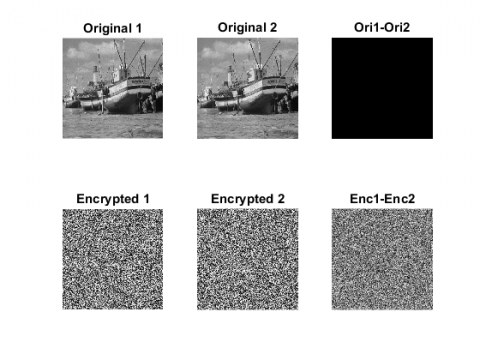Figure 12. The proposed scheme encrypts boat image twice utilizing the same set of security keys

6. Conclusion

In this paper, an improved classical Logistic map is suggested to enhance the chaotic features in order to protect digital images in the transmission and storage. The experimental tests on the chaotic conduct and the chaotic ambit regarding the improved logistic map in terms of NIST test, Lyapunov exponent, bifurcation and comparison with the classical logistic map, illustrate better chaotic performance. Furthermore, a new tweakable image encryption algorithm based on confusion-diffusion architecture containing two tours of diffusion and permutation process is introduced. The tests results prove that the suggested algorithm is simple, efficient and has good execution in image encryption and the ability to withstand several attacks.

References

 Liu, X.B., Xiao, D., Huang, W., Liu, C. (2019). Quantum block image encryption based on arnold transform and sine chaotification model. IEEE Access, 7: 57188-57199. https://doi.org/10.1109/ACCESS.2019.2914184

 Zhou, N.R., Hu, Y.Q., Gong, L.H., Li, G.Y. (2017). Quantum image encryption scheme with iterative generalized Arnold transforms and quantum image cycle shift operations. Quantum Inf. Process., 16(6): 164. https://doi.org/10.1007/s11128-017-1612-0

 Wang, X.Y., Zhang, Y.Q., Bao, X.M. (2015). A novel chaotic image encryption scheme using DNA sequence operations. Opt. Lasers Eng., 73: 53-61. https://doi.org/10.1016/j.optlaseng.2015.03.022

 Liu, H.J., Wang, X.Y., Kadir, A. (2012). Image encryption using DNA complementary rule and chaotic maps. Applied Soft Computing, 12(5): 1457-1466. https://doi.org/10.1016/j.asoc.2012.01.016

 Slimane, N.B., Aouf, N., Bouallegue, K., Machhout, M. (2018). A novel chaotic image cryptosystem based on DNA sequence operations and single neuron model. Multimedia Tools and Applications, 77(23): 30993-31019. https://doi.org/10.1007/s11042-018-6145-8

 Zhang, X.Q., Wang, X.S. (2017). Multiple-image encryption algorithm based on mixed image element and permutation. Optics and Lasers in Engineering, 92: 6-16. https://doi.org/10.1016/j.optlaseng.2016.12.005

 Zahmoul, R., Ejbali, R., Zaied, M. (2017). Image encryption based on new Beta chaotic maps. Optics and Lasers in Engineering, 96: 39-49. https://doi.org/10.1016/j.optlaseng.2017.04.009

 Herbadji, D., Derouiche, N., Belmeguenai, A., Bekkouche, T., Labiad, A., Lashab, M., Herbadji, A. (2018). A new image encryption scheme using an enhanced logistic map. 2018 Int. Conf. on Applied Smart Systems (ICASS), Medea, Algeria, pp. 1-6. https://doi.org/10.1109/ICASS.2018.8652065

 Abanda, Y., Tiedeu, A. (2016). Image encryption by chaos mixing. IET Image Processing, 10(10): 742-750. 2016. https://doi.org/10.1049/iet-ipr.2015.0244

 Hua, Z.Y., Zhou, Y.C., Huang, H.J. (2019). Cosine-transform-based chaotic system for image encryption. Information Science (Ny), 480: 403-419. https://doi.org/10.1016/j.ins.2018.12.048

 Liu, H.J., Wang, X.Y. (2010). Color image encryption based on one-time keys and robust chaotic maps. Computers & Mathematics with Applications, 59(10): 3320-3327. https://doi.org/10.1016/j.camwa.2010.03.017

 Liu, H.J., Wang, X.Y. (2011). Color image encryption using spatial bit-level permutation and high-dimension chaotic system. Optics Communications, 284(16-17): 3895-3903. https://doi.org/10.1016/j.optcom.2011.04.001

 Hua, Z.Y., Xu, B.X., Jin, F., Huang, H.J. (2019). Image encryption using josephus problem and filtering diffusion. IEEE Access, 7: 8660-8674. https://doi.org/10.1109/ACCESS.2018.2890116

 Wang, X.Y., Yang, L., Liu, R., Kadir, A. (2010). A chaotic image encryption algorithm based on perceptron model. Nonlinear Dynamics, 62(3): 615-621. https://doi.org/10.1007/s11071-010-9749-8

 Wang, X.Y., Feng, L., Zhao, H.Y. (2019). Fast image encryption algorithm based on parallel computing system. Information Science (Ny)., 486: 340-358. https://doi.org/10.1016/j.ins.2019.02.049

 Dridi, M., Hajjaji, M.A., Bouallegue, B., Mtibaa, A. (2016). Cryptography of medical images based on a combination between chaotic and neural network. IET Image Processing, 10(11): 830-839. https://doi.org/10.1049/iet-ipr.2015.0868

 Khan, J.S., Ahmad, J. (2019). Chaos based efficient selective image encryption. Multidimensional Systems and Signal Processing, 30(2): 943-961. https://doi.org/10.1007/s11045-018-0589-x

 Arroyo, D., Diaz, J., Rodriguez, F.B. (2013). Cryptanalysis of a one round chaos-based substitution permutation network. Signal Processing, 93(5): 1358-1364. https://doi.org/10.1016/j.sigpro.2012.11.019

 Kumar, R.R., Kumar, M.B. (2014). A new chaotic image encryption using parametric switching based permutation and diffusion. ICTACT J. Image Video Process, 4(4). https://doi.org/10.21917/ijivp.2014.0114

 Zhu, H.G., Zhao, Y.R., Song, Y.J. (2019). 2D logistic-modulated-sine-coupling-logistic chaotic map for image encryption. IEEE Access, 7: 14081-14098. https://doi.org/10.1109/ACCESS.2019.2893538

 Hua, Z.Y., Zhou, B.H., Zhou, Y.C. (2018). Sine chaotification model for enhancing chaos and its hardware implementation. IEEE Transactions on Industrial Electronics, 66(2): 1273-1284. https://doi.org/10.1109/TIE.2018.2833049

 Hua, Z.Y., Zhou, B.H., Zhou, Y.C. (2017). Sine-transform-based chaotic system with FPGA implementation. IEEE Transactions on Industrial Electronics, 65(3): 2557-2566. https://doi.org/10.1109/TIE.2017.2736515

 Liskov, M., Rivest, R.L., Wagner, D. (2002). Tweakable block ciphers. Annual International Cryptology Conference, pp. 31-46. https://doi.org/10.1007/3-540-45708-9_3

 Özkaynak, F. (2015). A novel method to improve the performance of chaos based evolutionary algorithms. Optik, 126(24): 5434-5438. https://doi.org/10.1016/j.ijleo.2015.09.098

 Ramadan, N., Ahmed, H.E.H., Elkhamy, S.E., El-Samie, F.E.A. (2016). Chaos-based image encryption using an improved quadratic chaotic map. American Journal of Signal Processing, 6(1): 1-13. https://doi.org/10.5923/j.ajsp.20160601.01

 Wang, X., Wang, S., Zhang,Y., Guo, K. (2017). A novel image encryption algorithm based on chaotic shuffling method. Information Security Journal: A Global Perspective, 26(1): 7-16. https://doi.org/10.1080/19393555.2016.1272725

 Hua, Z.Y., Zhou, Y.C. (2017). Design of image cipher using block-based scrambling and image filtering. Information Science (Ny), 396: 97-113. https://doi.org/10.1016/j.ins.2017.02.036

 Hua, Z.Y., Zhou, Y.C., Pun, C.M., Chen, C.L.P. (2015). 2D Sine Logistic modulation map for image encryption. Information Science (Ny), 297: 80-94. https://doi.org/10.1016/j.ins.2014.11.018

 Zhang, Y.Q., Wang, X.Y. (2015). A new image encryption algorithm based on non-adjacent coupled map lattices. Applied Soft Computing, 26: 10-20. https://doi.org/10.1016/j.asoc.2014.09.039

 Wang, X.Y., Liu, L.T., Zhang, Y.Q. (2015). A novel chaotic block image encryption algorithm based on dynamic random growth technique. Optics and Lasers in Engineering, 66: 10-18. https://doi.org/10.1016/j.optlaseng.2014.08.005

 Zhang, Y.Q., Wang, X.Y. (2014). A symmetric image encryption algorithm based on mixed linear--nonlinear coupled map lattice. Information Science (Ny), 273: 329-351. https://doi.org/10.1016/j.ins.2014.02.156

 Chai, X.L., Gan, Z.H., Yuan, K., Chen, Y.R., Liu, X.X. (2019). A novel image encryption scheme based on DNA sequence operations and chaotic systems. Neural Computing and Applications, 31(1): 219-237. https://doi.org/10.1007/s00521-017-2993-9

 Bashir, Z., Rashid, T., Zafar, S. (2016). Hyperchaotic dynamical system based image encryption scheme with time-varying delays. Pacific Science Review A: Natural Science and Engineering, 18(3): 254-260. https://doi.org/10.1016/j.psra.2016.11.003

 Abbasi, S.F., Ahmad, J., Khan, J.S., Khan, M.A., Sheikh, S.A. (2018). Visual meaningful encryption scheme using intertwinning logistic map. Science and Information Conference, pp. 764-773. https://doi.org/10.1007/978-3-030-01177-2_56

 Khan, J.S., Ahmad, J., Abbasi, S.F., Arshad, Kayhan, S.K. (2018). DNA sequence based medical image encryption scheme. 2018 10th Computer Science and Electronic Engineering (CEEC), pp. 24-29. https://doi.org/10.1109/CEEC.2018.8674221

 Mondal, B., Singh, S., Kumar, P. (2019). A secure image encryption scheme based on cellular automata and chaotic skew tent map. Journal of Information Security and Applications, 45:. 117-130. https://doi.org/10.1016/j.jisa.2019.01.010

 Hamza, R., Titouna, F. (2016). A novel sensitive image encryption algorithm based on the Zaslavsky chaotic map. Information Security Journal: A Global Perspective, 25(4-6): 162-179. https://doi.org/10.1080/19393555.2016.1212954